### Home > PC > Chapter 8 > Lesson 8.1.4 > Problem8-56

8-56.
1. Write out the first four terms of the following sequences, then evaluate the limits. Homework Help ✎

1.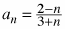. Then find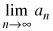.

2.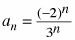. Then find.

3.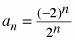. Then find.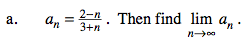$a_1=\frac{2-1}{3+1}=?$

$a_2=\frac{2-2}{3+2}=?$

$a_3=\frac{2-3}{3+3}=?$

$a_4=\frac{2-4}{3+4}=?$

1. Split the fraction into two fractions:

$a_n=\frac{2}{3+n}-\frac{n}{3+n}$

2. Use the dominant term idea to find the limit.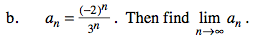$a_1=\frac{(-2)^1}{3^1}=?$

$a_2=\frac{(-2)^2}{3^2}=?$

$a_3=\frac{(-2)^3}{3^3}=?$

$a_4=\frac{(-2)^4}{3^4}=?$

The dominant term is in the denominator.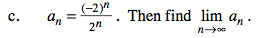$a_1=\frac{(-2)^1}{2^1}=?$

$a_2=\frac{(-2)^2}{2^2}=?$

$a_3=\frac{(-2)^3}{2^3}=?$

$a_4=\frac{(-2)^4}{2^4}=?$

The dominant term is in both the denominator and the numerator.